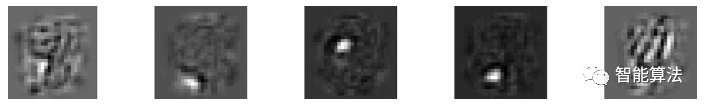# 深度学习算法(第29期)----可视化自编码器和无监督预训练

2019/10/04 11:50

#### 可视化重构

n_test_digits = 2X_test = mnist.test.images[:n_test_digits]with tf.Session() as sess:    [...] # Train the Autoencoder    outputs_val = outputs.eval(feed_dict={X: X_test})def plot_image(image, shape=[28, 28]):    plt.imshow(image.reshape(shape), cmap="Greys", interpolation="nearest")    plt.axis("off")for digit_index in range(n_test_digits):    plt.subplot(n_test_digits, 2, digit_index * 2 + 1)    plot_image(X_test[digit_index])    plt.subplot(n_test_digits, 2, digit_index * 2 + 2)    plot_image(outputs_val[digit_index])

#### 可视化特征

with tf.Session() as sess:    [...] # train autoencoder    weights1_val = weights1.eval()for i in range(5):    plt.subplot(1, 5, i + 1)    plot_image(weights1_val.T[I])#### 无监督预训练0
0 收藏

### 作者的其它热门文章0 评论
0 收藏
0